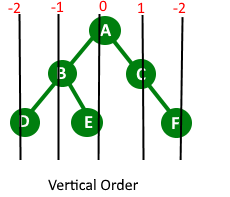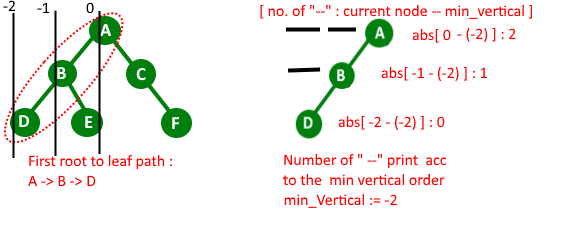Related Articles

# Print all root to leaf paths with there relative positions

• Difficulty Level : Medium
• Last Updated : 04 Sep, 2021

Given a binary tree, print the root to the leaf path, but add “_” to indicate the relative position.

Example:

```Input : Root of below tree
A
/   \
B      C
/ \    / \
D   E   F  G

Output : All root to leaf paths
_ _ A
_ B
D

_ A
B
_ E

A
_ C
F

A
_ C
_ _ G```

The idea base on print path in vertical orderBelow is complete algorithm :
1) We do Preorder traversal of the given Binary Tree. While traversing the tree, we can recursively calculate horizontal distances or HDs. We initially pass the horizontal distance as 0 for root. For left subtree, we pass the Horizontal Distance as Horizontal distance of root minus 1. For right subtree, we pass the Horizontal Distance as Horizontal Distance of root plus 1. For every HD value, we maintain a list of nodes in a vector (” that will store information of current node horizontal distance and key value of root “).we also maintain the order of node (order in which they appear in path from root to leaf). for maintaining the order,here we used vector.

2) While we reach to leaf node during traverse we print that path with underscore “_”

Print_Path_with_underscore function……a) First find the minimum Horizontal distance of the current path.
……b) After that we traverse current path
……….First Print number of underscore “_” : abs (current_node_HD – minimum-HD)
……….Print current node value.
We do this process for all root to leaf path

Below is the implementation of the above idea.

## C++

 `// C++ program to print all root to leaf paths``// with there relative position``#include``using` `namespace` `std;` `#define MAX_PATH_SIZE 1000` `// tree structure``struct` `Node``{``    ``char` `data;``    ``Node *left, *right;``};` `// function create new node``Node * newNode(``char` `data)``{``    ``struct` `Node *temp = ``new` `Node;``    ``temp->data = data;``    ``temp->left = temp->right = NULL;``    ``return` `temp;``}` `// store path information``struct` `PATH``{``    ``int`  `Hd; ``// horizontal distance of node from root.``    ``char` `key; ``// store key``};` `// Prints given root to leaf path with underscores``void` `printPath(vector < PATH > path, ``int` `size)``{``    ``// Find the minimum horizontal distance value``    ``// in current root to leaf path``    ``int` `minimum_Hd = INT_MAX;` `    ``PATH p;` `    ``// find minimum horizontal distance``    ``for` `(``int` `it=0; it &AllPath,``                       ``int` `HD, ``int` `order )``{``    ``// base case``    ``if``(root == NULL)``        ``return``;` `    ``// leaf node``    ``if` `(root->left == NULL && root->right == NULL)``    ``{``        ``// add leaf node and then print path``        ``AllPath[order] = (PATH { HD, root->data });``        ``printPath(AllPath, order+1);``        ``return``;``    ``}` `    ``// store current path information``    ``AllPath[order] = (PATH { HD, root->data });` `    ``// call left sub_tree``    ``printAllPathsUtil(root->left, AllPath, HD-1, order+1);` `    ``//call left sub_tree``    ``printAllPathsUtil(root->right, AllPath, HD+1, order+1);``}` `void` `printAllPaths(Node *root)``{``    ``// base case``    ``if` `(root == NULL)``        ``return``;` `    ``vector Allpaths(MAX_PATH_SIZE);``    ``printAllPathsUtil(root, Allpaths, 0, 0);``}` `// Driver program to test above function``int` `main()``{``    ``Node *root = newNode(``'A'``);``    ``root->left = newNode(``'B'``);``    ``root->right = newNode(``'C'``);``    ``root->left->left = newNode(``'D'``);``    ``root->left->right = newNode(``'E'``);``    ``root->right->left = newNode(``'F'``);``    ``root->right->right = newNode(``'G'``);``    ``printAllPaths(root);``    ``return` `0;``}`

## Java

 `// Java program to print all root to leaf``// paths with there relative position``import` `java.util.ArrayList;` `class` `Graph{``    ` `static` `final` `int` `MAX_PATH_SIZE = ``1000``;` `// tree structure``static` `class` `Node``{``    ``char` `data;``    ``Node left, right;``};` `// Function create new node``static` `Node newNode(``char` `data)``{``    ``Node temp = ``new` `Node();``    ``temp.data = data;``    ``temp.left = temp.right = ``null``;``    ``return` `temp;``}` `// Store path information``static` `class` `PATH``{``    ` `    ``// Horizontal distance of node from root.``    ``int` `Hd;``    ` `    ``// Store key``    ``char` `key;` `    ``public` `PATH(``int` `Hd, ``char` `key)``    ``{``        ``this``.Hd = Hd;``        ``this``.key = key;``    ``}` `    ``public` `PATH()``    ``{}``};` `// Prints given root to leaf path with underscores``static` `void` `printPath(ArrayList path, ``int` `size)``{``    ` `    ``// Find the minimum horizontal distance value``    ``// in current root to leaf path``    ``int` `minimum_Hd = Integer.MAX_VALUE;` `    ``PATH p;` `    ``// Find minimum horizontal distance``    ``for``(``int` `it = ``0``; it < size; it++)``    ``{``        ``p = path.get(it);``        ``minimum_Hd = Math.min(minimum_Hd, p.Hd);``    ``}` `    ``// Print the root to leaf path with "_"``    ``// that indicate the related position``    ``for``(``int` `it = ``0``; it < size; it++)``    ``{``        ` `        ``// Current tree node``        ``p = path.get(it);``        ``int` `noOfUnderScores = Math.abs(``            ``p.Hd - minimum_Hd);` `        ``// Print underscore``        ``for``(``int` `i = ``0``; i < noOfUnderScores; i++)``            ``System.out.print(``"_"``);` `        ``// Print current key``        ``System.out.println(p.key);``    ``}``    ``System.out.println(``"=============================="``);``}` `// A utility function print all path from root to leaf``// working of this function is similar to function of``// "Print_vertical_order" : Print paths of binary tree``// in vertical order``// https://www.geeksforgeeks.org/print-binary-tree-vertical-order-set-2/``static` `void` `printAllPathsUtil(Node root,``                              ``ArrayList AllPath,``                              ``int` `HD, ``int` `order)``{``    ` `    ``// Base case``    ``if` `(root == ``null``)``        ``return``;``        ` `    ``// Leaf node``    ``if` `(root.left == ``null` `&& root.right == ``null``)``    ``{``        ` `        ``// Add leaf node and then print path``        ``AllPath.set(order, ``new` `PATH(HD, root.data));``        ``// AllPath[order] = (PATH { HD, root.data });``        ``printPath(AllPath, order + ``1``);``        ``return``;``    ``}``    ` `    ``// Store current path information``    ``AllPath.set(order, ``new` `PATH(HD, root.data));``    ``// AllPath[order] = (PATH { HD, root.data });` `    ``// Call left sub_tree``    ``printAllPathsUtil(root.left, AllPath,``                      ``HD - ``1``, order + ``1``);` `    ``// Call left sub_tree``    ``printAllPathsUtil(root.right, AllPath,``                      ``HD + ``1``, order + ``1``);``}` `static` `void` `printAllPaths(Node root)``{``    ` `    ``// Base case``    ``if` `(root == ``null``)``        ``return``;` `    ``ArrayList Allpaths = ``new` `ArrayList<>();``    ``for``(``int` `i = ``0``; i < MAX_PATH_SIZE; i++)``    ``{``        ``Allpaths.add(``new` `PATH());``    ``}``    ``printAllPathsUtil(root, Allpaths, ``0``, ``0``);``}` `// Driver code``public` `static` `void` `main(String[] args)``{``    ``Node root = newNode(``'A'``);``    ``root.left = newNode(``'B'``);``    ``root.right = newNode(``'C'``);``    ``root.left.left = newNode(``'D'``);``    ``root.left.right = newNode(``'E'``);``    ``root.right.left = newNode(``'F'``);``    ``root.right.right = newNode(``'G'``);``    ` `    ``printAllPaths(root);``}``}` `// This code is contributed by sanjeev2552`

## Python3

 `# Python3 program to print the longest``# leaf to leaf path` `# Tree node structure used in the program``MAX_PATH_SIZE ``=` `1000` `class` `Node:``    ` `    ``def` `__init__(``self``, x):``        ``self``.data ``=` `x``        ``self``.left ``=` `None``        ``self``.right ``=` `None` `# Prints given root to leafAllpaths``# with underscores``def` `printPath(size):``    ` `    ``global` `Allpaths``    ` `    ``# Find the minimum horizontal distance``    ``# value in current root to leafAllpaths``    ``minimum_Hd ``=` `10``*``*``19` `    ``p ``=` `[]` `    ``# Find minimum horizontal distance``    ``for` `it ``in` `range``(size):``        ``p ``=` `Allpaths[it]``        ``minimum_Hd ``=` `min``(minimum_Hd, p[``0``])` `    ``# Print the root to leafAllpaths with "_"``    ``# that indicate the related position``    ``for` `it ``in` `range``(size):``        ` `        ``# Current tree node``        ``p ``=` `Allpaths[it]``        ``noOfUnderScores ``=` `abs``(p[``0``] ``-` `minimum_Hd)` `        ``# Print underscore``        ``for` `i ``in` `range``(noOfUnderScores):``            ``print``(end ``=` `"_ "``)` `        ``# Print current key``        ``print``(p[``1``])``        ` `    ``print``(``"=============================="``)` `# A utility function prall path from root to leaf``# working of this function is similar to function of``# "Print_vertical_order" : Prpaths of binary tree``# in vertical order``# https://www.geeksforgeeks.org/print-binary-tree-vertical-order-set-2/``def` `printAllPathsUtil(root, HD, order):``    ` `    ``# Base case``    ``global` `Allpaths` `    ``if` `(root ``=``=` `None``):``        ``return` `    ``# Leaf node``    ``if` `(root.left ``=``=` `None` `and` `root.right ``=``=` `None``):``        ` `        ``# Add leaf node and then prpath``        ``Allpaths[order] ``=` `[HD, root.data]``        ``printPath(order ``+` `1``)``        ``return` `    ``# Store current path information``    ``Allpaths[order] ``=` `[HD, root.data]` `    ``# Call left sub_tree``    ``printAllPathsUtil(root.left, HD ``-` `1``, order ``+` `1``)` `    ``# Call left sub_tree``    ``printAllPathsUtil(root.right, HD ``+` `1``, order ``+` `1``)` `def` `printAllPaths(root):``    ` `    ``global` `Allpaths``    ` `    ``# Base case``    ``if` `(root ``=``=` `None``):``        ``return` `    ``printAllPathsUtil(root, ``0``, ``0``)` `# Driver code``if` `__name__ ``=``=` `'__main__'``:``    ` `    ``Allpaths ``=` `[ [``0``, ``0``]  ``for` `i ``in` `range``(MAX_PATH_SIZE)]` `    ``root ``=` `Node(``'A'``)``    ``root.left ``=` `Node(``'B'``)``    ``root.right ``=` `Node(``'C'``)``    ``root.left.left ``=` `Node(``'D'``)``    ``root.left.right ``=` `Node(``'E'``)``    ``root.right.left ``=` `Node(``'F'``)``    ``root.right.right ``=` `Node(``'G'``)` `    ``printAllPaths(root)` `# This code is contributed by mohit kumar 29`

## C#

 `// C# program to print all root to leaf``// paths with there relative position``using` `System;``using` `System.Collections.Generic;``public``  ``class` `Graph``  ``{` `    ``static` `readonly` `int` `MAX_PATH_SIZE = 1000;` `    ``// tree structure``    ``public``      ``class` `Node``      ``{``        ``public``          ``char` `data;``        ``public``          ``Node left, right;``      ``};` `    ``// Function create new node``    ``static` `Node newNode(``char` `data)``    ``{``      ``Node temp = ``new` `Node();``      ``temp.data = data;``      ``temp.left = temp.right = ``null``;``      ``return` `temp;``    ``}` `    ``// Store path information``    ``public` `      ``class` `PATH``      ``{` `        ``// Horizontal distance of node from root.``        ``public``          ``int` `Hd;` `        ``// Store key``        ``public` `          ``char` `key;``        ``public` `PATH(``int` `Hd, ``char` `key)``        ``{``          ``this``.Hd = Hd;``          ``this``.key = key;``        ``}``        ``public` `PATH()``        ``{}``      ``};` `    ``// Prints given root to leaf path with underscores``    ``static` `void` `printPath(List path, ``int` `size)``    ``{` `      ``// Find the minimum horizontal distance value``      ``// in current root to leaf path``      ``int` `minimum_Hd = ``int``.MaxValue;``      ``PATH p;` `      ``// Find minimum horizontal distance``      ``for``(``int` `it = 0; it < size; it++)``      ``{``        ``p = path[it];``        ``minimum_Hd = Math.Min(minimum_Hd, p.Hd);``      ``}` `      ``// Print the root to leaf path with "_"``      ``// that indicate the related position``      ``for``(``int` `it = 0; it < size; it++)``      ``{` `        ``// Current tree node``        ``p = path[it];``        ``int` `noOfUnderScores = Math.Abs(``          ``p.Hd - minimum_Hd);` `        ``// Print underscore``        ``for``(``int` `i = 0; i < noOfUnderScores; i++)``          ``Console.Write(``"_"``);` `        ``// Print current key``        ``Console.WriteLine(p.key);``      ``}``      ``Console.WriteLine(``"=============================="``);``    ``}` `    ``// A utility function print all path from root to leaf``    ``// working of this function is similar to function of``    ``// "Print_vertical_order" : Print paths of binary tree``    ``// in vertical order``    ``// https://www.geeksforgeeks.org/print-binary-tree-vertical-order-set-2/``    ``static` `void` `printAllPathsUtil(Node root,``                                  ``List AllPath,``                                  ``int` `HD, ``int` `order)``    ``{` `      ``// Base case``      ``if` `(root == ``null``)``        ``return``;` `      ``// Leaf node``      ``if` `(root.left == ``null` `&& root.right == ``null``)``      ``{` `        ``// Add leaf node and then print path``        ``AllPath[order] = ``new`  `PATH(HD, root.data);` `        ``// AllPath[order] = (PATH { HD, root.data });``        ``printPath(AllPath, order + 1);``        ``return``;``      ``}` `      ``// Store current path information``      ``AllPath[order]= ``new` `PATH(HD, root.data);` `      ``// AllPath[order] = (PATH { HD, root.data });` `      ``// Call left sub_tree``      ``printAllPathsUtil(root.left, AllPath,``                        ``HD - 1, order + 1);` `      ``// Call left sub_tree``      ``printAllPathsUtil(root.right, AllPath,``                        ``HD + 1, order + 1);``    ``}` `    ``static` `void` `printAllPaths(Node root)``    ``{` `      ``// Base case``      ``if` `(root == ``null``)``        ``return``;` `      ``List Allpaths = ``new` `List();``      ``for``(``int` `i = 0; i < MAX_PATH_SIZE; i++)``      ``{``        ``Allpaths.Add(``new` `PATH());``      ``}``      ``printAllPathsUtil(root, Allpaths, 0, 0);``    ``}` `    ``// Driver code``    ``public` `static` `void` `Main(String[] args)``    ``{``      ``Node root = newNode(``'A'``);``      ``root.left = newNode(``'B'``);``      ``root.right = newNode(``'C'``);``      ``root.left.left = newNode(``'D'``);``      ``root.left.right = newNode(``'E'``);``      ``root.right.left = newNode(``'F'``);``      ``root.right.right = newNode(``'G'``);` `      ``printAllPaths(root);``    ``}``  ``}` `// This code is contributed by aashish1995`

## Javascript

 ``

Output:

```_ _ A
_ B
D
==============================
_ A
B
_ E
==============================
A
_ C
F
==============================
A
_ C
_ _ G```

This article is contributed by Nishant Singh. If you like GeeksforGeeks and would like to contribute, you can also write an article using write.geeksforgeeks.org or mail your article to review-team@geeksforgeeks.org. See your article appearing on the GeeksforGeeks main page and help other Geeks.